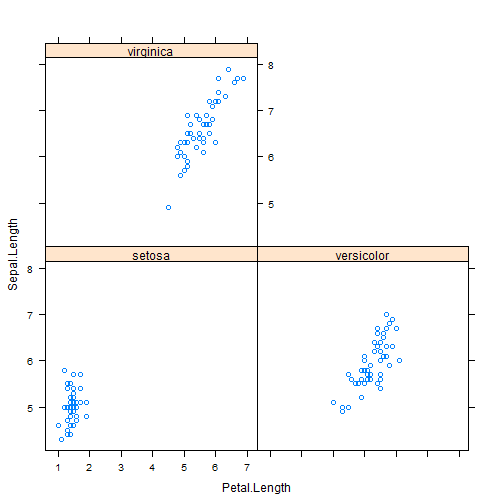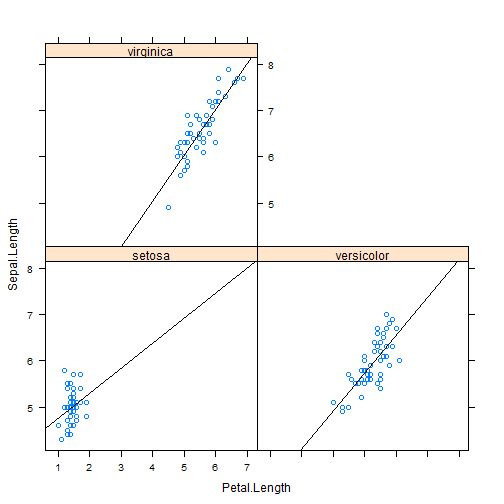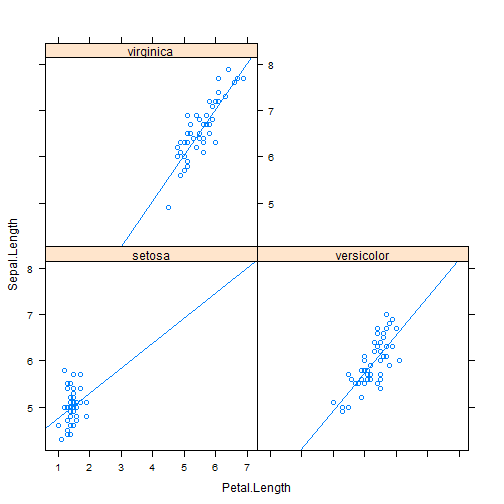# Add regression line using xyplot

| category RStudy  | tag R  Plot

### scatterplot

~x means display numeric variable x alone.

 **y ~ x A** means display the relationship between numeric variables y and x for each level of factor A.
 **y ~ x A*B** means display the relationship between numeric variables y and x separately for every combination of factor A and B levels.
head(iris)

Sepal.Length Sepal.Width Petal.Length Petal.Width Species
1          5.1         3.5          1.4         0.2  setosa
2          4.9         3.0          1.4         0.2  setosa
3          4.7         3.2          1.3         0.2  setosa
4          4.6         3.1          1.5         0.2  setosa
5          5.0         3.6          1.4         0.2  setosa
6          5.4         3.9          1.7         0.4  setosa

table(iris\$Species)


setosa versicolor  virginica
50         50         50


library(lattice)
xyplot(Sepal.Length ~ Petal.Length | Species, data = iris)xyplot(Sepal.Length ~ Petal.Length | Species, data = iris, panel = function(x,
y, ...) {
panel.xyplot(x, y, ...)
panel.lmline(x, y, ...)
})### Add regression line using type()

xyplot(Sepal.Length ~ Petal.Length | Species, data = iris, type = c("p", "r"))The type = argument can be used to enhance the figure with data-responsive elements. The default value type = “p”, which requests only points, type = “r” request a simple linear regression.